# User:IssaRice/Logical induction notation

Term Notation Type Definition Notes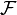$\mathcal F$-combination$A$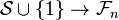$\mathcal S \cup \{1\} \to \mathcal F_n$ Function application of an$\mathcal F$-combination uses square brackets instead of parentheses. Why? As far as I can tell, this is because each coefficient is in$\mathcal F$ so is itself a function. This means we have two senses of "application": we can pick out the specific coefficient we want (square brackets), or we can apply each coefficient to return something (parentheses).
Holdings from$T$ against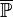$\overline{\mathbb P}$ (a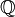$\mathbb Q$-combination)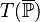$T(\overline{\mathbb P})$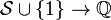$\mathcal S \cup \{1\} \to \mathbb Q$
Trading strategy$T$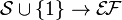$\mathcal S \cup \{1\} \to \mathcal{E\!F}$
Feature$\alpha$$[0,1]^{\mathcal S \times \mathbb N^{+}} \to \mathbb R$ or equivalently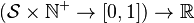$(\mathcal S \times \mathbb N^{+} \to [0,1]) \to \mathbb R$ or equivalently$\mathcal F$

Example of a 5-strategy given on p. 18 of the paper: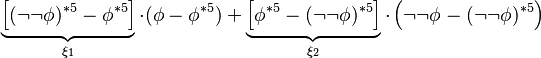$\underbrace{\left[(\neg\neg\phi)^{*5} -\phi^{*5}\right]}_{\xi_1} \cdot (\phi - \phi^{*5}) + \underbrace{\left[\phi^{*5} - (\neg \neg \phi)^{*5}\right]}_{\xi_2} \cdot \left(\neg\neg\phi - (\neg\neg\phi)^{*5}\right)$

Since the coefficients (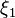$\xi_1$ and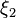$\xi_2$) are in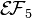$\mathcal{E\!F}_5$, this is an$\mathcal{E\!F}_5$-combination. Let's call this 5-strategy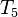$T_5$. We can pick out the coefficient for the$\phi$ term like$T_5[\phi] = (\neg\neg\phi)^{*5} -\phi^{*5}$. But since each coefficient is a feature (which is a function), we can also apply each coefficient to some valuation sequence$\overline{\mathbb V}$, like this: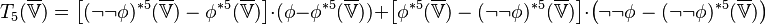$T_5(\overline{\mathbb V}) = \left[(\neg\neg\phi)^{*5}(\overline{\mathbb V}) -\phi^{*5}(\overline{\mathbb V})\right] \cdot (\phi - \phi^{*5}(\overline{\mathbb V})) + \left[\phi^{*5}(\overline{\mathbb V}) - (\neg \neg \phi)^{*5}(\overline{\mathbb V})\right] \cdot \left(\neg\neg\phi - (\neg\neg\phi)^{*5}(\overline{\mathbb V})\right)$

Now each coefficient is a real number, so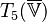$T_5(\overline{\mathbb V})$ is an$\mathbb R$-combination. Note that since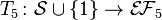$T_5\colon \mathcal S \cup \{1\} \to \mathcal{E\!F}_5$ is a function that takes a sentence or the number$1$ and$\overline{\mathbb V}$ is a valuation sequence (not a sentence or number), there appears to be a type error in writing$T_5(\overline{\mathbb V})$. What is going on is that we aren't evaluating$T_5$ at$\overline{\mathbb V}$; rather, we are evaluating each coefficient of$T_5$, to convert the range of$T_5$ from$\mathcal{E\!F}_5$ to$\mathbb R$.

To summarize the types:

•$T_5 \colon \mathcal S \cup \{1\} \to \mathcal{E\!F}_5$
•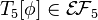$T_5[\phi] \in \mathcal{E\!F}_5$ in other words$T_5[\phi] \colon [0,1]^{\mathcal S\times \mathbb N^{+}} \to \mathbb R$
•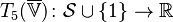$T_5(\overline{\mathbb V}) \colon \mathcal S \cup \{1\} \to \mathbb R$

If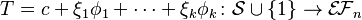$T = c + \xi_1\phi_1 + \cdots + \xi_k\phi_k \colon \mathcal S \cup \{1\} \to \mathcal{E\!F}_n$, then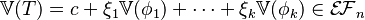$\mathbb V(T) = c + \xi_1\mathbb V(\phi_1) + \cdots + \xi_k\mathbb V(\phi_k) \in \mathcal{E\!F}_n$

and$T(\overline{\mathbb V}) = c(\overline{\mathbb V})+ \xi_1(\overline{\mathbb V})\phi_1 + \cdots + \xi_k(\overline{\mathbb V})\phi_k \colon \mathcal S \cup \{1\} \to \mathbb R$

and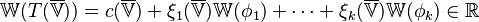$\mathbb W(T(\overline{\mathbb V})) = c(\overline{\mathbb V})+ \xi_1(\overline{\mathbb V})\mathbb W(\phi_1) + \cdots + \xi_k(\overline{\mathbb V})\mathbb W(\phi_k) \in \mathbb R$

I think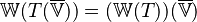$\mathbb W(T(\overline{\mathbb V})) = (\mathbb W(T))(\overline{\mathbb V})$ but the former notation seems to be preferred in the paper.